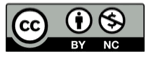# Python 正则表达式里的单行s和多行m模式

2014-08-18Python 的 re 模块内置函数几乎都有一个 flags 参数，规定了正则匹配时的各种策略模式，其中有两个模式：单行（re.DOTALL, 或者re.S）和多行（re.MULTILINE, 或者re.M）模式。本文简单介绍下这两种模式的用法和使用场合。

## 文件的保存形式：字节流

cat
dog

``````\$ xxd -b a.txt
0000000: 01100011 01100001 01110100 00001010 01100100 01101111  cat.do
0000006: 01100111 00001010                                      g.
``````

``````\$ xxd a
0000000: 6361 740a 646f 670a                      cat.dog.
``````

Linux环境下， `0a` 就是换行符，在编程语言里用 `\n` 表示，不同操作系统下的换行符有少许不同，见这篇文章 Python 换行符和多行模式

## 普通模式

In the default mode, this matches any character except a newline.

This is the first line.
This is the second line.
This is the third line.

``````>>> a = 'This is the first line.\nThis is the second line.\nThis is the third line.'
>>> print a
This is the first line.
This is the second line.
This is the third line.
>>> import re
>>> p = re.match(r'This.*line\.' ,a)
>>> p.group(0)
'This is the first line.'
>>>
``````

## 单行模式 re.DOTALL

If the DOTALL flag has been specified, this matches any character including a newline.

``````>>> q = re.match(r'This.*line\.', a, flags=re.DOTALL)
>>> q.group(0)
'This is the first line.\nThis is the second line.\nThis is the third line.'
``````

## 多行模式 re.MULTILINE

``````>>> a = 'This is the first line.\nThis is the second line.\nThis is the third line.'
>>> print a
This is the first line.
This is the second line.
This is the third line.
>>> import re
>>> re.findall(r'^This.*line\.\$', a)
[]
>>>
``````

``````>>> re.findall(r'^This.*line\.\$', a, flags=re.DOTALL)
['This is the first line.\nThis is the second line.\nThis is the third line.']
>>>
``````

``````> re.findall(r'^This.*?line\.\$', a, flags=re.DOTALL)
['This is the first line.\nThis is the second line.\nThis is the third line.']
>
``````

By default, ‘^’ matches only at the beginning of the string, and ‘\$’ only at the end of the string and immediately before the newline (if any) at the end of the string.

Python 文档中这么描述：

When specified, the pattern character ‘^’ matches at the beginning of the string and at the beginning of each line (immediately following each newline); and the pattern character ‘\$’ matches at the end of the string and at the end of each line (immediately preceding each newline).

``````> re.findall(r'^This.*line\.\$', a, flags=re.MULTILINE)
['This is the first line.', 'This is the second line.', 'This is the third line.']
``````

## 精彩评论